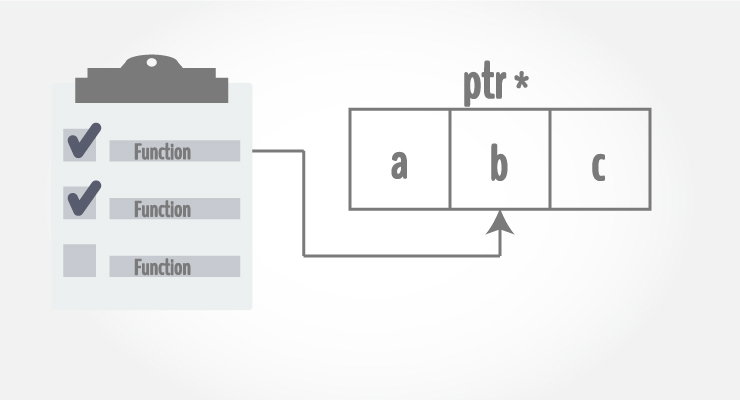# C++ Call by Reference: Using pointers [With Examples]

In this article, you'll learn to pass pointers as an argument to the function, and use it efficiently in your program.In C++ Functions article, you learned about passing arguments to a function. This method used is called passing by value because the actual value is passed.

However, there is another way of passing an argument to a function where where the actual value of the argument is not passed. Instead, only the reference to that value is passed.

## Example 1: Passing by reference without pointers

``````#include <iostream>
using namespace std;

// Function prototype
void swap(int&, int&);

int main()
{
int a = 1, b = 2;
cout << "Before swapping" << endl;
cout << "a = " << a << endl;
cout << "b = " << b << endl;

swap(a, b);

cout << "\nAfter swapping" << endl;
cout << "a = " << a << endl;
cout << "b = " << b << endl;

return 0;
}

void swap(int& n1, int& n2) {
int temp;
temp = n1;
n1 = n2;
n2 = temp;
}``````

Output

```Before swapping
a = 1
b = 2

After swapping
a = 2
b = 1```

In `main()`, two integer variables a and b are defined. And those integers are passed to a function `swap()` by reference.

Compiler can identify this is pass by reference because function definition is `void swap(int& n1, int& n2)` (notice the & sign after data type).

Only the reference (address) of the variables a and b are received in the `swap()` function and swapping takes place in the original address of the variables.

In the swap() function, n1 and n2 are formal arguments which are actually same as variables a and b respectively.

There is another way of doing this same exact task using pointers.

## Example 2: Passing by reference using pointers

``````#include <iostream>
using namespace std;

// Function prototype
void swap(int*, int*);

int main()
{
int a = 1, b = 2;
cout << "Before swapping" << endl;
cout << "a = " << a << endl;
cout << "b = " << b << endl;

swap(&a, &b);

cout << "\nAfter swapping" << endl;
cout << "a = " << a << endl;
cout << "b = " << b << endl;
return 0;
}

void swap(int* n1, int* n2) {
int temp;
temp = *n1;
*n1 = *n2;
*n2 = temp;
}``````

The output of this example is same as before.

In this case, the address of variable is passed during function call rather than the variable itself.

`swap(&a, &b); // &a is address of a and &b is address of b`

Since the address is passed instead of value, dereference operator must be used to access the value stored in that address.

```void swap(int* n1, int* n2) {
... .. ...
}
```

The `*n1` and `*n2` gives the value stored at address n1 and n2 respectively.

Since n1 contains the address of a, anything done to `*n1` changes the value of a in `main()` function as well. Similarly, b will have same value as `*n2`.